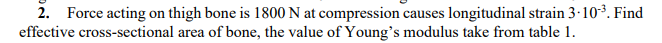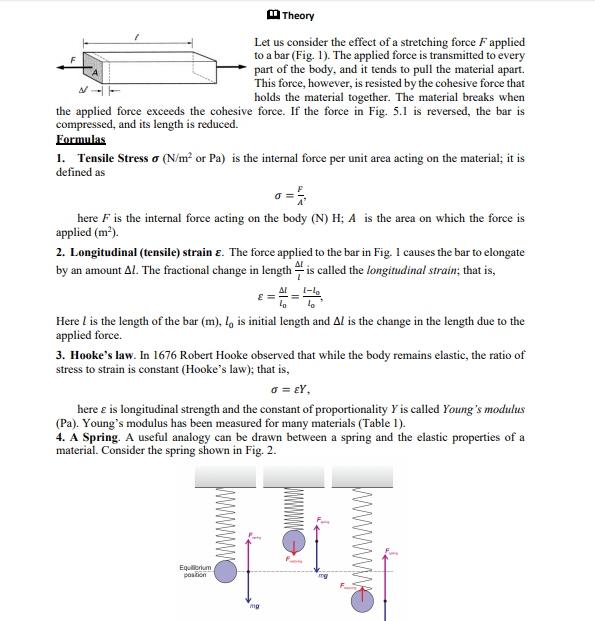# 2. Force acting on thigh bone is 1800 N at compression causes longitudinal strain 3-103. Findeffective cross-sectional area of bone, the value of Young's modulus take from table 1. TheoryLet us consider the effect of a stretching force F appliedto a bar (Fig. 1). The applied force is transmitted to everypart of the body, and it tends to pull the material apart.This force, however, is resisted by the cohesive force thatholds the material together. The material breaks whenthe applied force exceeds the cohesive force. If the force in Fig. 5.1 is reversed, the bar iscompressed, and its length is reduced.Formulas1. Tensile Stress o (N/m² or Pa) is the internal force per unit area acting on the material; it isdefined ashere F is the internal force acting on the body (N) H; A is the area on which the force isapplied (m²).2. Longitudinal (tensile) strain ɛ. The force applied to the bar in Fig. 1 causes the bar to elongateby an amount Al. The fractional change in length is called the longitudinal strain; that is,Al1-l.Here l is the length of the bar (m), l, is initial length and Al is the change in the length due to theapplied force.3. Hooke's law. In 1676 Robert Hooke observed that while the body remains elastic, the ratio ofstress to strain is constant (Hooke's law); that is,o = eY,here e is longitudinal strength and the constant of proportionality Y is called Young's modulus(Pa). Young's modulus has been measured for many materials (Table 1).4. A Spring. A useful analogy can be drawn between a spring and the elastic properties ofmaterial. Consider the spring shown in Fig. 2.Equilioriumpostionmg

Question
1 viewshelp_outlineImage Transcriptionclose2. Force acting on thigh bone is 1800 N at compression causes longitudinal strain 3-103. Find effective cross-sectional area of bone, the value of Young's modulus take from table 1. fullscreenhelp_outlineImage TranscriptioncloseTheory Let us consider the effect of a stretching force F applied to a bar (Fig. 1). The applied force is transmitted to every part of the body, and it tends to pull the material apart. This force, however, is resisted by the cohesive force that holds the material together. The material breaks when the applied force exceeds the cohesive force. If the force in Fig. 5.1 is reversed, the bar is compressed, and its length is reduced. Formulas 1. Tensile Stress o (N/m² or Pa) is the internal force per unit area acting on the material; it is defined as here F is the internal force acting on the body (N) H; A is the area on which the force is applied (m²). 2. Longitudinal (tensile) strain ɛ. The force applied to the bar in Fig. 1 causes the bar to elongate by an amount Al. The fractional change in length is called the longitudinal strain; that is, Al 1-l. Here l is the length of the bar (m), l, is initial length and Al is the change in the length due to the applied force. 3. Hooke's law. In 1676 Robert Hooke observed that while the body remains elastic, the ratio of stress to strain is constant (Hooke's law); that is, o = eY, here e is longitudinal strength and the constant of proportionality Y is called Young's modulus (Pa). Young's modulus has been measured for many materials (Table 1). 4. A Spring. A useful analogy can be drawn between a spring and the elastic properties of material. Consider the spring shown in Fig. 2. Equiliorium postion mg fullscreen
check_circle

Step 1
Step 2

### Want to see the full answer?

See Solution

#### Want to see this answer and more?

Solutions are written by subject experts who are available 24/7. Questions are typically answered within 1 hour.*

See Solution
*Response times may vary by subject and question.
Tagged in

### Other